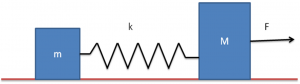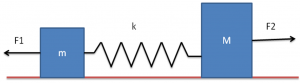# Two Block Spring System

Here we will understand the mechanism of the two block-spring system. We will look at an experiment and understand all the related terms as well as learn to solve some problems.

## Two-Block Spring System Experiment And Mechanism

A block of mass m is connected to another block of mass M by a massless spring of spring constant k. The blocks are kept on a smooth horizontal plane. At first, the blocks are at rest and the spring is unstretched when a constant force F starts acting on the block of mass M to pull it. Now we have to find the maximum extension of the spring.Taking the two blocks plus the spring as a system:

Since there is an external force F on the system, there will be some acceleration of the centre of mass, which can be calculated by the formula:

$\overrightarrow{a_{cm}} = \frac {\overrightarrow{F_{ext}}}{M}$

where,

$\overrightarrow{F_{ext}}$   is the net external force on the system

M is the total mass of the system

a = $\frac {F}{m ~+~ M}$

Now, let us solve this problem from the frame of reference of a centre of mass. This means that we would have to apply a pseudo force ma towards left of the block of mass m and another pseudo force Ma towards left on the block of mass M.

Net external force on the block of mass m is

F1 = ma =  $\frac {mF}{m + M}$

This force will be in the left direction

Net external force on the block of mass M is

F2 = F – Ma

= F –  $\frac {MF}{m + M} = \frac {mF}{m~+ M}$

This force will be in the right direction

The situation from the frame of the centre of mass would be as shown in the picture below:The centre of mass is at rest in this frame, the blocks will move in opposite directions and after some time, when the extension of the spring is maximum, they will come to rest for an instant. Let the block of mass m moves a distance x1 and the block of mass M moves a distance x2. The total work done by the forces F1 and F2 will be:

W = F1 x1 + F2 x2

=  $\frac {mF}{m + M} ( x_1 + x_2)$

This work done should be equal to the change in potential energy of the spring because the change in kinetic energy will be zero from the frame of the centre of mass

$\frac{mF}{m+M} (x_1+x_2) = \frac 12 k ( x_1+x_2)^2$

Or

$( x_1+x_2) = \frac {2 mF}{k ( m+M)}$

If you want to learn more about spring block systems, get connected to our mentors here at BYJU’s classes.